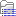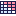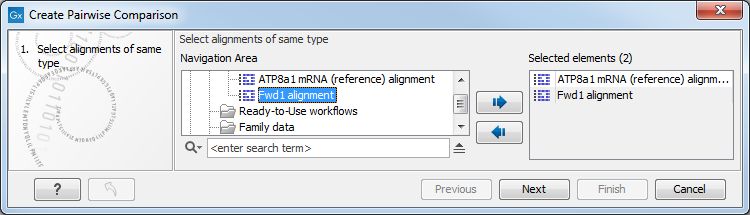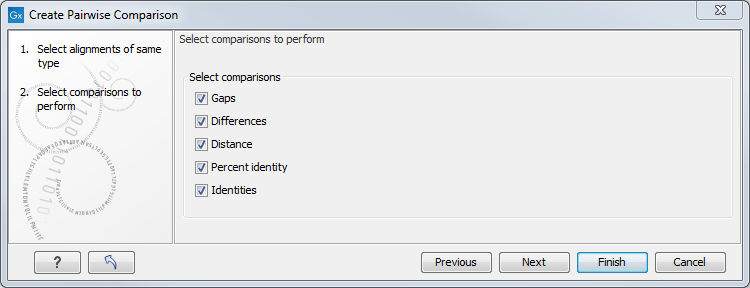# Pairwise comparison

For a given set of aligned sequences it is possible to make a pairwise comparison in which each pair of sequences are compared to each other. This provides an overview of the diversity among the sequences in the alignment.

In CLC Genomics Workbench this is done by creating a comparison table:

Toolbox | Classical Sequence Analysis () | Alignments and Trees ()| Create Pairwise Comparison ()

This opens the dialog displayed in figure 20.16:Figure 20.16: Creating a pairwise comparison table.

Select at least two alignments alignment to compare. A pairwise comparison can also be performed for a selected part of an alignment:

right-click on an alignment selection | Pairwise Comparison ()

There are five kinds of comparison that can be made between the sequences in the alignment, as shown in figure 20.17.Figure 20.17: Adjusting parameters for pairwise comparison.

• Gaps Calculates the number of alignment positions where one sequence has a gap and the other does not.
• Identities Calculates the number of identical alignment positions to overlapping alignment positions between the two sequences. An overlapping alignment position is a position where at least one residue is present, rather than only gaps.
• Differences Calculates the number of alignment positions where one sequence is different from the other. This includes gap differences as in the Gaps comparison.
• Distance Calculates the Jukes-Cantor distance between the two sequences. This number is given as the Jukes-Cantor correction of the proportion between identical and overlapping alignment positions between the two sequences.
• Percent identity Calculates the percentage of identical residues in alignment positions to overlapping alignment positions between the two sequences.

Subsections